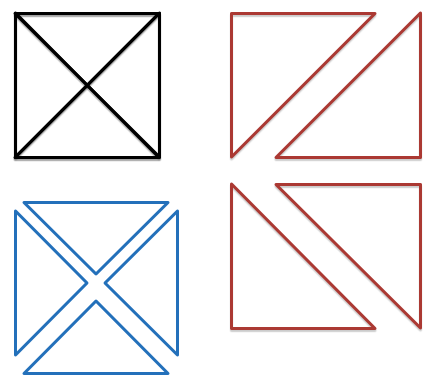# Triangles in a Complete Graph

Probability Level 3A straight-line drawing of a graph $G$ is a drawing of $G$ on the plane such that all its edges are straight line segments.

The maximum number of triangles that can be found in a straight-line drawing of the complete graph $K_4$ is 8, as seen in the image. For $K_5$, there can be up to 35 triangles.

What is the maximum number of triangles that can be found in a straight-line drawing of the complete graph $K_{10}$?

×